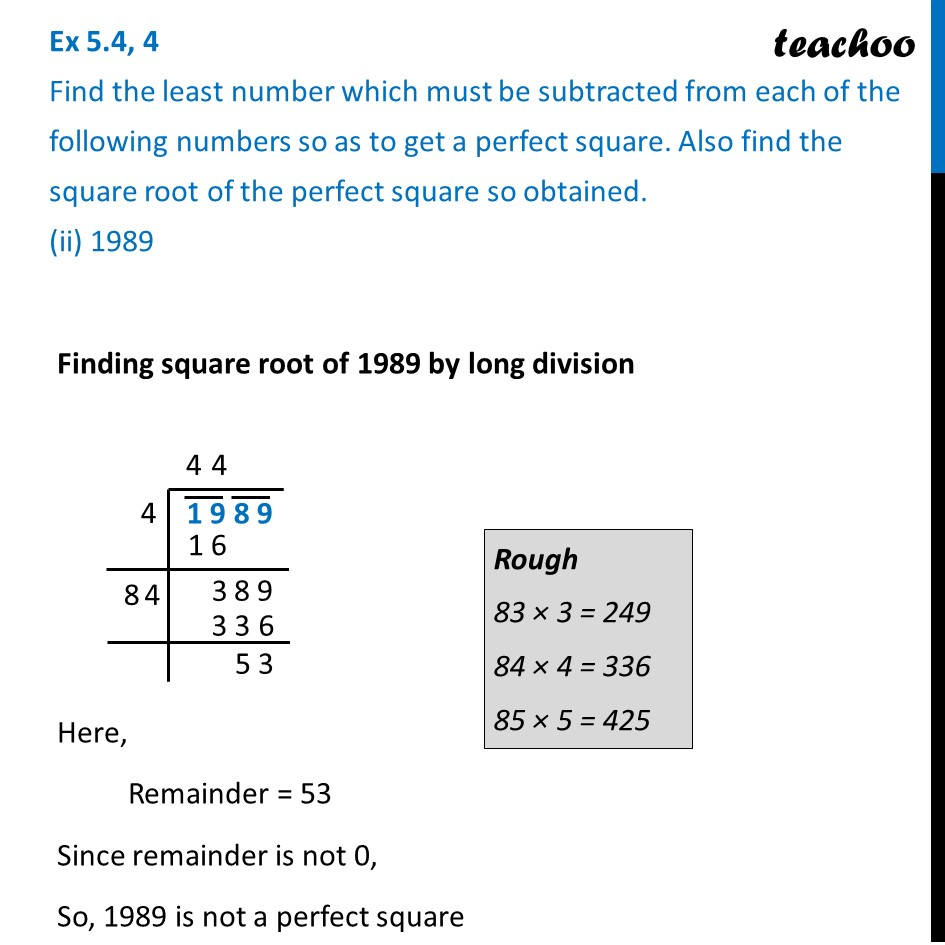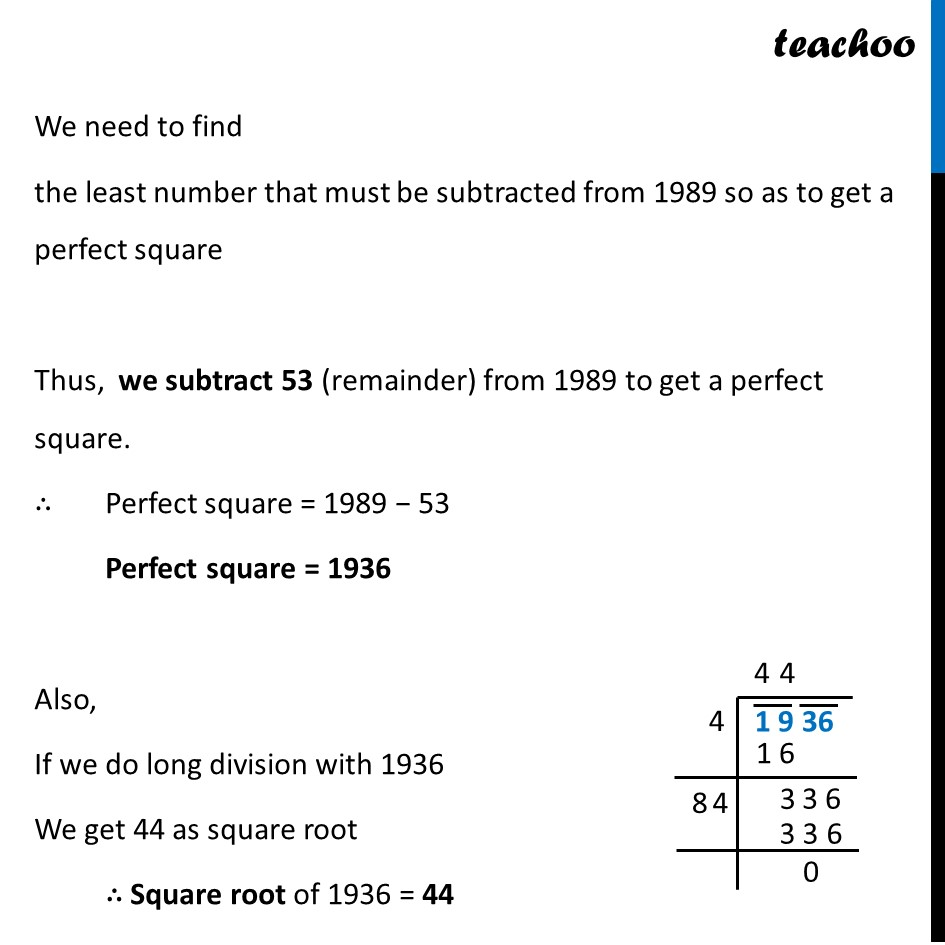Ex 5.4

Chapter 5 Class 8 Squares and Square Roots
Serial order wiseLearn in your speed, with individual attention - Teachoo Maths 1-on-1 Class

### Transcript

Ex 5.4, 4 Find the least number which must be subtracted from each of the following numbers so as to get a perfect square. Also find the square root of the perfect square so obtained. (ii) 1989 Finding square root of 1989 by long division Here, Remainder = 53 Since remainder is not 0, So, 1989 is not a perfect square We need to find the least number that must be subtracted from 1989 so as to get a perfect square Thus, we subtract 53 (remainder) from 1989 to get a perfect square. ∴ Perfect square = 1989 − 53 Perfect square = 1936 Also, If we do long division with 1936 We get 44 as square root ∴ Square root of 1936 = 44